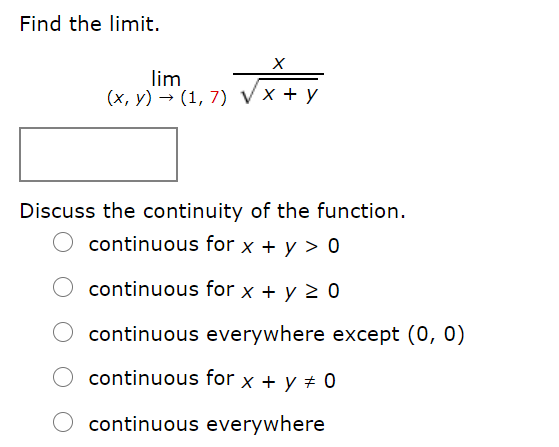# Find the limit. lim (x, y) → (1, 7) Vx + y Discuss the continuity of the function. continuous for x + y > 0 continuous for x + y 2 0 continuous everywhere except (0, 0) continuous for x + y ± 0 continuous everywhere

Question

Calculus 3 Question

Section 13.2help_outlineImage TranscriptioncloseFind the limit. lim (x, y) → (1, 7) Vx + y Discuss the continuity of the function. continuous for x + y > 0 continuous for x + y 2 0 continuous everywhere except (0, 0) continuous for x + y ± 0 continuous everywhere fullscreen

### Want to see this answer and more?

Experts are waiting 24/7 to provide step-by-step solutions in as fast as 30 minutes!*

*Response times vary by subject and question complexity. Median response time is 34 minutes and may be longer for new subjects.
Tagged in
MathCalculus

### Limits